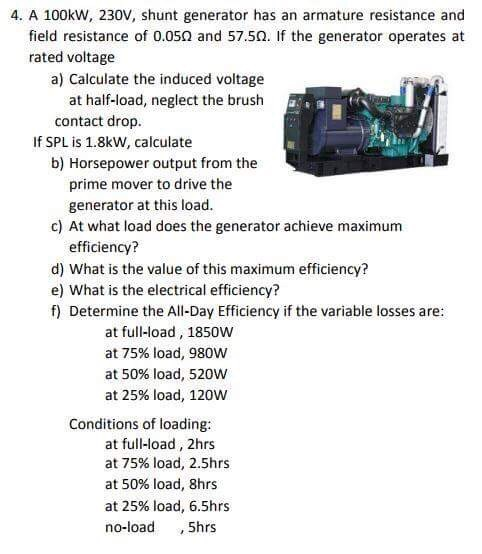### Create an Account

Home / Questions / 4. A 100kw, 230V, shunt generator has an armature resistance and field resistance of 0.05O and 57.5O

# 4. A 100kw, 230V, shunt generator has an armature resistance and field resistance of 0.05O and 57.5O4. A 100kw, 230V, shunt generator has an armature resistance and field resistance of 0.05Ω and 57.5Ω. If the generator operates at rated voltage a) Calculate the induced voltage contact drop b) Horsepower output from the at half-load, neglect the brush fィ If SPL is 1.8kW, calculate prime mover to drive the generator at this load c) At what load does the generator achieve maximum d) What is the value of this maximum efficiency? f) Determine the All-Day Efficiency if the variable losses are efficiency? e) What is the electrical efficiency? at full-load, 1850W at 75% load, 980W at 50% load, 520w at 25% load, 120W Conditions of loading at full-load, 2hrs at 75% load, 2.5hrs at 50% load, 8hrs at 25% load, 6.5hrs no-load , 5hrs

Apr 06 2020 View more View LessSubscribe To Get Solution